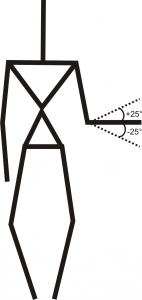Posted on

# Opened and closed hand gesture detectionWe detect the gesture of the opened and closed hand with sensor Kinect. State of the hand was divided into 2 parts, when it is opened (palm) or closed (fist). We assume that hand is rotated in a parallel way with the sensor and is captured her profile.

Functions used:

## The process

1. Get point in the middle of the hand and limit around her window
```Point pointHand(handFrameSize.width, handFrameSize.height);
Rect rectHand = Rect(pos - pointHand, pos + pointHand);
Mat depthExtractTemp = depthImageGray(rectHand); //extract hand image from depth image
Mat depthExtract(handFrameSize.height * 2, handFrameSize.width * 2, CV_8UC1);
```

2. Find the minimum depth value in the window
```int tempDepthValue = getMinValue16(depthExtractTemp);
```
3. Convert window from 16bit to 8bit Â and use as mean value of the minimum depth
```ImageExtractDepth(&amp;depthExtractTemp, &amp;depthExtract, depthValue );
```

4. Cut half hand in the window
1. for the right hand from the center to the right
2. for the left hand from the center to the left
5. Use thresholding, create mask and cut distant hand (finger)
```Mat depthThresh;
threshold( depthThresh, depthThresh, 180, 255, CV_THRESH_BINARY_INV);
```

6. Determine the size of the rectangle surrounding this part of the hand
```Mat depthExtract2;
morphologyEx(depthExtract2, depthExtract2, MORPH_CLOSE, structElement3);
vector<vector<Point>> contours;
vector<Vec4i> hierarchy;
findContours(depthExtract2, contours, hierarchy, CV_RETR_EXTERNAL, CV_CHAIN_APPROX_SIMPLE, cvPoint(0,0));
fitEllipse(Mat(contours[i]));
```
7. If aspect ratio of width and height of the rectangle is greater than 1, then hand is opened, else hand is closed

## Limitation

• Maximal distance detection is 2 meters
• Maximal slope hand is up or down by 25 degrees
• Profile of hand must be turned parallel with the sensor## Result

Detection of both hand (right and left) takes 4ms.